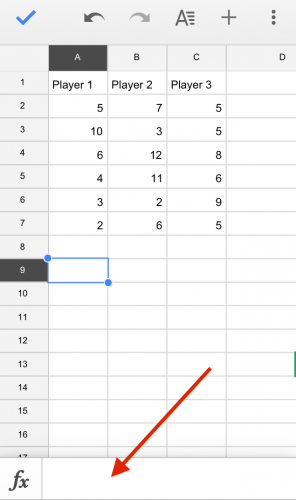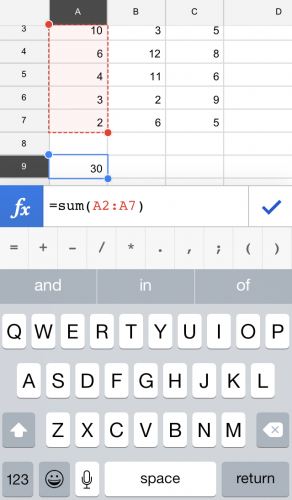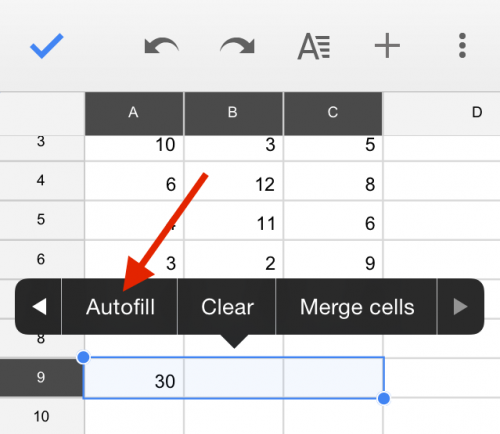HomeInternetAndroidHow to Sum a Column in Google Sheets Mobile

# How to Sum a Column in Google Sheets Mobile

#### One of the most common uses of spreadsheets is adding up lists of numbers. This is done by using the “Sum” formula. It is quick and easy and can be copied over multiple columns.

1.Enter your data. Tap the cell where you want to sum up your data, and then tap the formula bar on the bottom of the screen.2.Next, type the following:

=sum(

Then, select the range you want to sum. The easiest way to do this is to tap a cell in the range, and then drag a corner to highlight the whole target range in red. Type in a ). The total formula should look like this:

=sum(A2:A7)

Note that the cells you are summing do not have to be in the same column. You can tap to select each cell manually that you want to add together. If you use this method, Sheets will separate each cell reference with a comma instead of using a colon to show a consecutive range. This would look something like this:

=sum(A2, A3, B2, B3, C2, C7)

Click the check mark to exit out of entry mode. The cell will now display the sum of the cells you selected.3.Perhaps you have multiple columns that you want to sum. This is easy to do with Autofill. Select the cell with the formula you just entered and the cells that you want to fill with the sum. Tap on the blue selected region, and tap the arrow to the right until you see Autofill. Select this option, and the cells will now contain a sum. Note that this used relative cell locations. This means that if the first cell contained a formula to sum rows 2 to 7 in column A, and you autofill this formula to columns B and C, the autofilled cells will contain the sum of rows 2 to 7 in columns B and C.RELATED ARTICLES